﻿ 基于MSR的水下图像增强算法研究

# 基于MSR的水下图像增强算法研究Underwater Image Enhancement Algorithm Based on Improved Retinex Method

Abstract: The paper aimed at improving the problem of color distortion, blur and underexposure, which caused by absorption of water and light scattering in the underwater environment, and uneven light caused by artificial illumination in the deep sea. According to the ideal of removing the color distortion after the image blurring removal, a novel method of image enhancement has propose, combining multi-scale retinex (MSR) and color correction algorithm based on color histogram quantization. The color correction algorithm based on color channel histogram was used to adjust the image to improve problems of the low contrast, color attenuation, overall fuzzy, unclear details and so on. The experimental results showed that the proposed method can effectively reduce the influence of color distortion and highlight the image details, so that the underwater color image has better visual effect.

1. 引言

1963年E. Land等人  提出了人类视觉的亮度和颜色感知的模型，即一种颜色恒常知觉的计算理论——Retinex理论。Retinex是一个合成词，它的构成是retina(视网膜) + cortex(皮层)→Retinex。(解释了同一物体在有着不同光谱分布和强度的光源照射下给人带来的色彩感知相对恒定的现象。)40多年来，J. J. McCann和D. J. Jobson、Zia-Ur Rahman、G. A. Woodell等人模仿人类视觉系统发展了Retinex算法，从单尺度Retinex算法(single scale retinex, SSR)改进成多尺度加权平均的Retinex算法(multi-scale retinex, MSR)，再发展成带彩色恢复的多尺度Retinex算法(multi-scale retinex with color restoration, MSRCR)。基于Retinex的方法已经应用到了彩色图像增强、雾图像滤波、水下图像以及航空图像处理等领域  。

2. MSR图像增强算法

2.1. Retinex理论

Retinex理论认为，一幅给定的图像 $S\left(x,y\right)$ 可以分解为两个不同的图像：反射图像 $R\left(x,y\right)$ 和入射图像(亮度图像) $L\left(x,y\right)$ ，入射光照在反射物体上，通过反射物的反射形成反射光进入人眼，就是人类所看到的图像，图像S是图像L和图像R的乘积。即：

$S\left(x,y\right)=R\left(x,y\right)×L\left(x,y\right)$ (1)

$\mathrm{log}\left[R\left(x,y\right)\right]=\mathrm{log}\left[S\left(x,y\right)\right]-\mathrm{log}\left[L\left(x,y\right)\right]$ (2)

$R\left(x,y\right)=\mathrm{exp}\left(\mathrm{log}\left(I\left(x,y\right)\right)-\mathrm{log}\left(I\left(x,y\right)\ast G\left(x,y\right)\right)\right)$ (3)

$G\left(x,y\right)=\lambda \cdot {\text{e}}^{\frac{-\left({x}^{2}+{y}^{2}\right)}{{c}^{2}}}$

2.2. MSR算法

${R}_{k}\left(x,y\right)=\underset{i=1}{\overset{3}{\sum }}{\omega }_{i}\left\{\mathrm{log}{S}_{k}\left(x,y\right)-\mathrm{log}\left[{F}_{i}\left(x,y\right)\ast {S}_{k}\left(x,y\right)\right]\right\}$ (4)

3. 颜色校正

$J{\left(x,y\right)}_{CR}=\left\{\begin{array}{l}{V}_{\mathrm{min}},\text{\hspace{0.17em}}\text{\hspace{0.17em}}\text{\hspace{0.17em}}\text{\hspace{0.17em}}\text{\hspace{0.17em}}\text{\hspace{0.17em}}\text{\hspace{0.17em}}\text{\hspace{0.17em}}\text{\hspace{0.17em}}\text{\hspace{0.17em}}\text{\hspace{0.17em}}\text{\hspace{0.17em}}\text{\hspace{0.17em}}\text{\hspace{0.17em}}\text{\hspace{0.17em}}\text{\hspace{0.17em}}\text{\hspace{0.17em}}\text{\hspace{0.17em}}\text{\hspace{0.17em}}\text{\hspace{0.17em}}\text{\hspace{0.17em}}\text{\hspace{0.17em}}\text{\hspace{0.17em}}\text{\hspace{0.17em}}\text{\hspace{0.17em}}\text{\hspace{0.17em}}\text{\hspace{0.17em}}\text{\hspace{0.17em}}\text{\hspace{0.17em}}\text{\hspace{0.17em}}J\left(x,y\right)<{V}_{\mathrm{min}}\\ \frac{255\left(J\left(x,y\right)-{V}_{\mathrm{min}}\right)}{{V}_{\mathrm{max}}-{V}_{\mathrm{min}}},\text{\hspace{0.17em}}\text{\hspace{0.17em}}\text{\hspace{0.17em}}\text{\hspace{0.17em}}{V}_{\mathrm{min}} (5)

4. 实验结果及分析Table 1. Quantitative comparison of Performance Indexes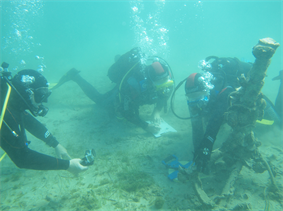原图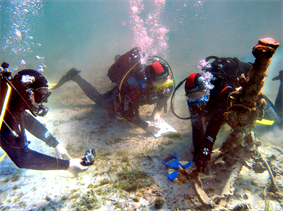GIMP结果文献6算法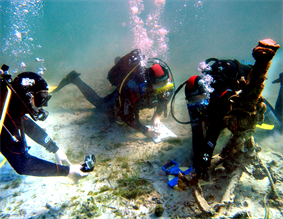本文算法

Figure 1. Three kinds of enhancement algorithm result of the first underwater image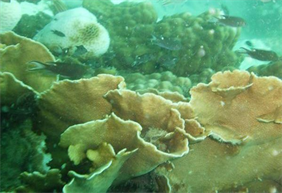原图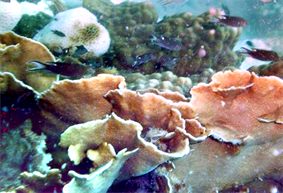GIMP结果文献6算法本文算法

Figure 2. Three kinds of enhancement algorithm result of the second underwater image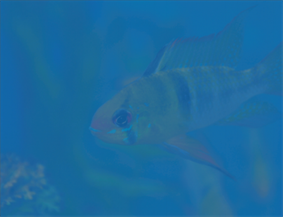原图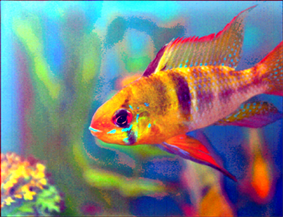GIMP结果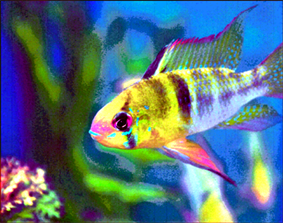文献6算法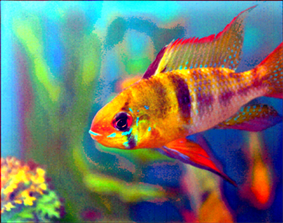本文算法

Figure 3. Three kinds of enhancement algorithm result of the third underwater image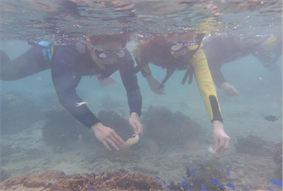原图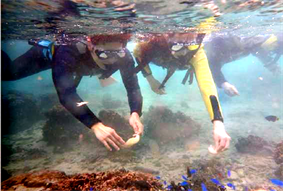GIMP结果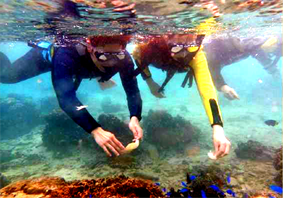文献6算法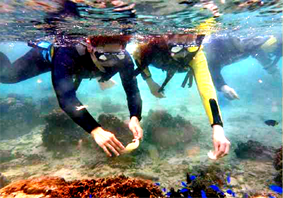本文算法

Figure 4. Three kinds of enhancement algorithm result of the forth underwater image

5. 结论

 徐岩, 马硕, 王权威. 一种利用前景模型的水下图像增强算法[J]. 小型微型计算机系统, 2017, 38(12): 2802-2806.

 Land, E.H. and Mc Cann, J.J. (1971) Lightness and Retinex Theory. Journal of the Optical Society of America, 61, 1- 11.
https://doi.org/10.1364/JOSA.61.000001

 Zhang, S., Wang, T., Dong, J.Y. and Yu, H. (2017) Underwater Image Enhancement via Extended Multi-Scale Retinex. Neurocomputing, 245, 1-9.
https://doi.org/10.1016/j.neucom.2017.03.029

 Wang, Y., Wang, H., Yin, C. and Dai, M. (2016) Biologically Inspired Image Enhancement Based on Retinex. Neurocomputing, 177, 373-384.
https://doi.org/10.1016/j.neucom.2015.10.124

 Jung, C., Sun, T. and Jiao, L. (2013) Eye Detection under Varying Illumination Using the Retinex Theory. Neurocomputing, 113, 130-137.
https://doi.org/10.1016/j.neucom.2013.01.038

 Joshi, K.R. and Kamathe, R.S. (2008) Quantification of Retinex in Enhancement of Weather Degraded Images. International Conference on Audio, Language and Image Processing (ICALIP 2008), July 7-9 2008, Shanghai, 1229-1233.
https://doi.org/10.1109/ICALIP.2008.4590120

 SM, A.R. and Supriya, M. (2015) Underwater Image Enhancement Using Single Scale Retinex on a Reconﬁgurable Hardware. 2015 IEEE International Symposium on Ocean Electronics (SYMPOL), 1-5.

 Fu, X.Y., Zhuang, P.X. and Liao, Y.H. (2014) A Retinex-Based Enhancement Approach for Single Underwater Image. Proceedings of IEEE International Conference on Image Processing, October 27-30 2014, Paris, 4572-4576.

 曾凡锋, 刘树鹏. Retinex在光照不均文本图像中的研究[J]. 计算机工程与设计, 2017, 38(11): 3072-3079.

 Rahman, Z., Jobson, D.J. and Woodell, G.A. (1996) Multi Scale Retinex for Color Image Enhancement. International Conferences on Proceedings of Image Processing, September 19-19 1996, Lausanne, 1103-1106.
https://doi.org/10.1109/ICIP.1996.560995

 Yu, T. and Wang, R. (2016) Scene Parsing Using Graph Matching on Street-View Data. Computer Vision and Image Understanding, 145, 70-80.
https://doi.org/10.1016/j.cviu.2016.01.004

 Tan, M., Hu, Z., Wang, B., Zhao, J. and Wang, Y. (2016) Robust Object Recognition via Weakly Supervised Metric and Template Learning. Neurocomputing, 181, 96-107.
https://doi.org/10.1016/j.neucom.2015.04.123

Top